# How to Count Cells that Contain even or odd numbers in Excel

This post will guide you how to count the number of cells that contain odd or even numbers within a range of cells using a formula in Excel 2013/2016.How do I count cells that contain odd numbers through the use of an Excel VBA Macro.

## Count Number of Cells that Contain Even Numbers

Assuming that you have a data list in the range of cells B1:B6, and you want count the number of cells containing only even numbers, and write the result in cell D1.You can use a formula based on the SUMPRODUCT function and the MOD function. Like this:

=SUMPRODUCT(–(MOD(B1:B6,2)=0))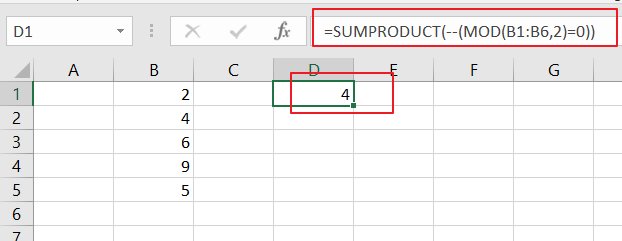Let’s see how this formula works:

=MOD(B1:B6,2)=0

The Mod function can be used to check whether the cell within the range B1:B6 containing the numbers if they are divided by 2 or not. If True, it indicates that that number is an even number. This formula returns an array result like:

{TRUE;TRUE;TRUE;FALSE;FALSE;TRUE}

The double negative operator would convert the above result array into 1 and 0. And still returns an array result. Like:

{1;1;1;0;0;1}

The SUMPRODUCT function will add those numbers in the array result and returns the final result.

## Count Number of Cells that Contain Odd Numbers

If you want to count number that contain odd numbers within a range of cells B1:B6, and you can use a formula based on the SUMPRODUCT function along with the MOD function. Like below:

=SUMPRODUCT(–(MOD(B1:B6,2)<>0))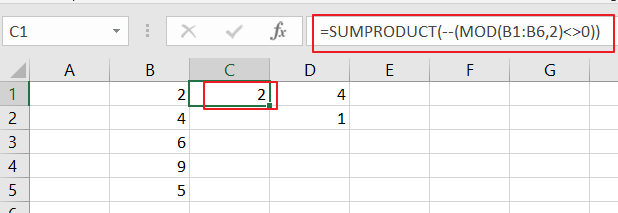The Mod function will check whether the cell with the range B1:B6 containing numbers are divided by 2 or not.

## Count Number of Cells that Contain Odd Numbers Using VBA

You can also count cells that contain odd numbers from a range using VBA macro, just do the following steps:

Step1# open your excel workbook and then click on “Visual Basic” command under DEVELOPER Tab, or just press “ALT+F11” shortcut.Step2#  then the “Visual Basic Editor” window will appear.

Step3# click “Insert” ->”Module” to create a new module.Step4# paste the below VBA code into the code window. Then clicking “Save” button.

```Sub CountCellsContainOddNumbers()
Dim mysheet As Worksheet
Dim myrange As Range
Set mysheet = Worksheets("Sheet3")
Set myrange = mysheet.Range("B1:B6")
For Each xcell In myrange
cellmod = xcell Mod 2
oddnum = oddnum + cellmod
Next xcell
mysheet.Range("D1") = oddnum

End Sub```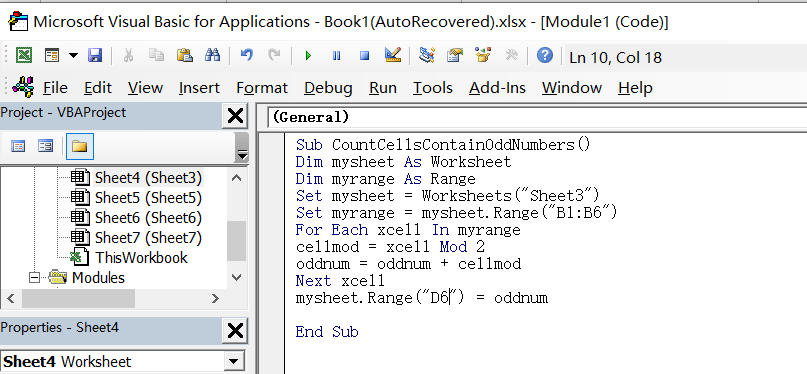Step5# back to the current worksheet, click on `Macros` button under `Code` group. then click `Run` button.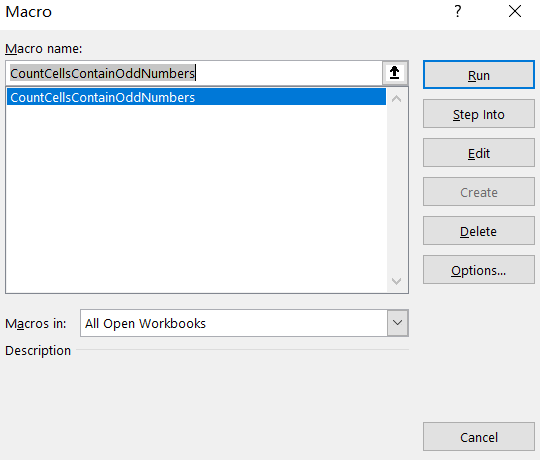Step6# let’s see the result: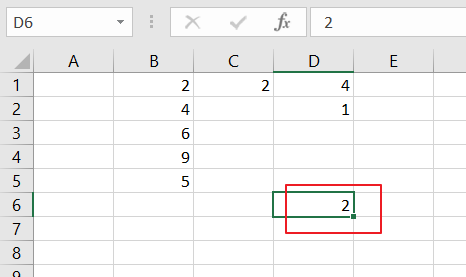### Related Functions

• Excel SUMPRODUCT function
The Excel SUMPRODUCT function multiplies corresponding components in the given one or more arrays or ranges, and returns the sum of those products. The syntax of the SUMPRODUCT function is as below:= SUMPRODUCT (array1,[array2],…)…
• Excel MOD function
he Excel MOD function returns the remainder of two numbers after division. So you can use the MOD function to get the remainder after a number is divided by a divisor in Excel. The syntax of the MOD function is as below:=MOD (number, divisor)….